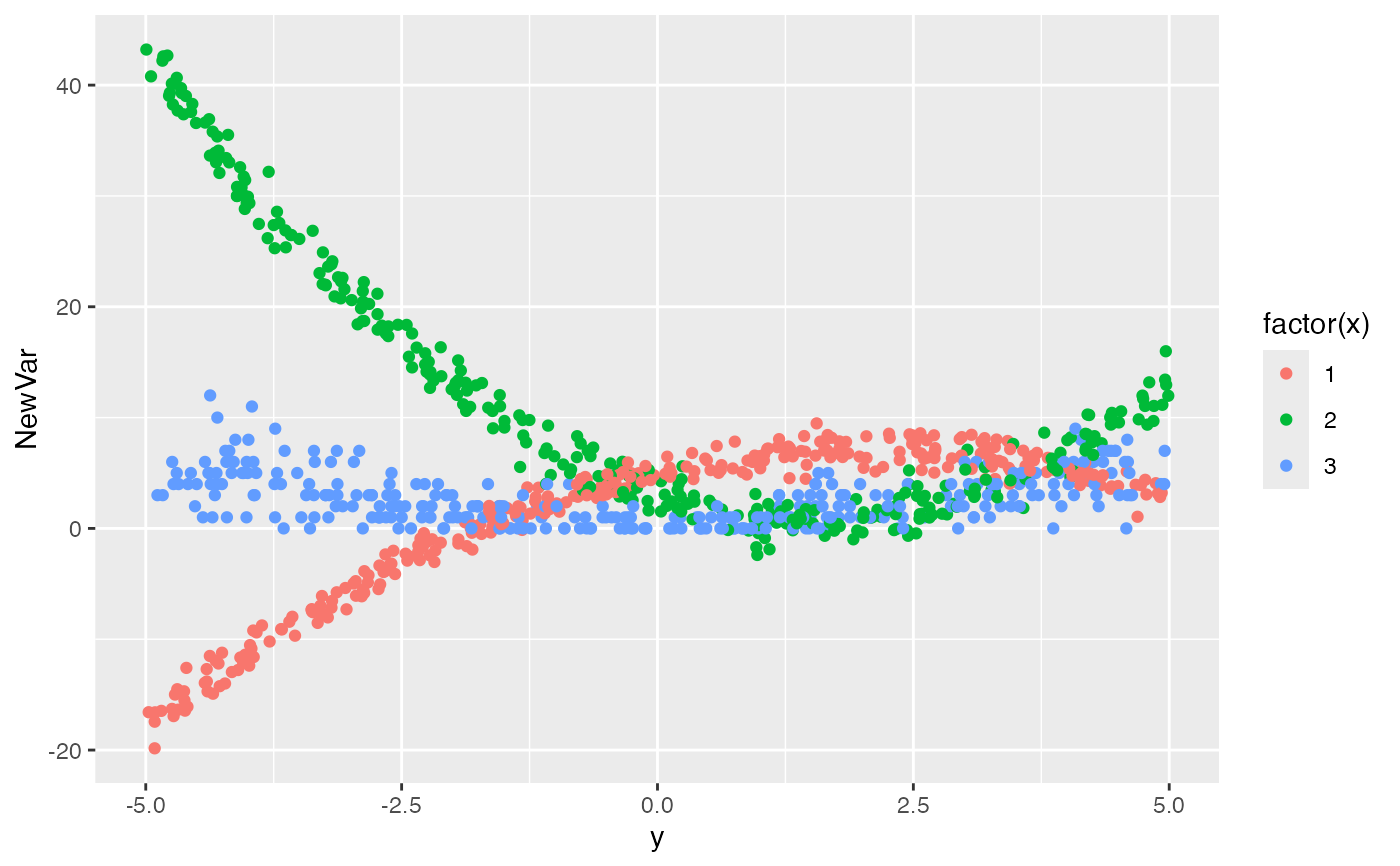Add a single column to existing data set based on a condition

addCondition(condDefs, dtOld, newvar, envir = parent.frame())

## Arguments

condDefs Name of definitions for added column Name of data table that is to be updated Name of new column to add Environment the data definitions are evaluated in. Defaults to base::parent.frame.

## Value

An updated data.table that contains the added simulated data

## Examples


# New data set

def <- defData(varname = "x", dist = "categorical", formula = ".33;.33")
def <- defData(def, varname = "y", dist = "uniform", formula = "-5;5")

dt <- genData(1000, def)
#> Warning: Probabilities do not sum to 1. Adding category to all rows!
# Define conditions

defC <- defCondition(
condition = "x == 1", formula = "5 + 2*y-.5*y^2",
variance = 1, dist = "normal"
)
defC <- defCondition(defC,
condition = "x == 2",
formula = "3 - 3*y + y^2", variance = 2, dist = "normal"
)
defC <- defCondition(defC,
condition = "x == 3",
formula = "abs(y)", dist = "poisson"
)